# Linear Search CS 5010 Program Design Paradigms Bootcamp

• Slides: 23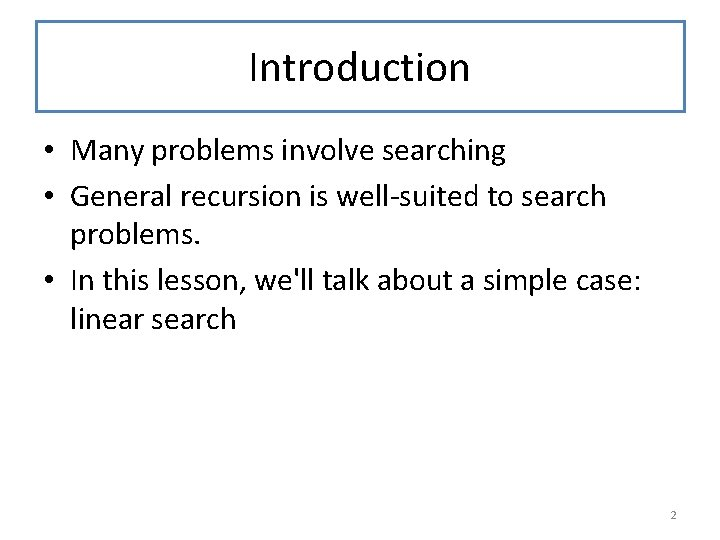Introduction • Many problems involve searching • General recursion is well-suited to search problems. • In this lesson, we'll talk about a simple case: linear search 2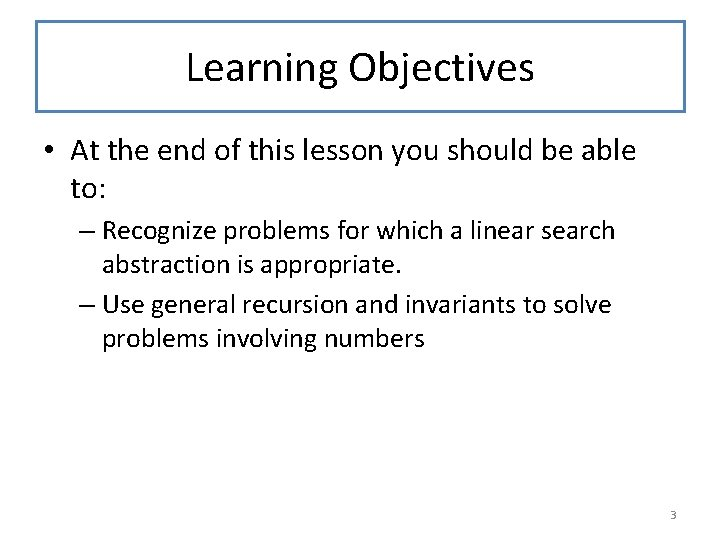Learning Objectives • At the end of this lesson you should be able to: – Recognize problems for which a linear search abstraction is appropriate. – Use general recursion and invariants to solve problems involving numbers 3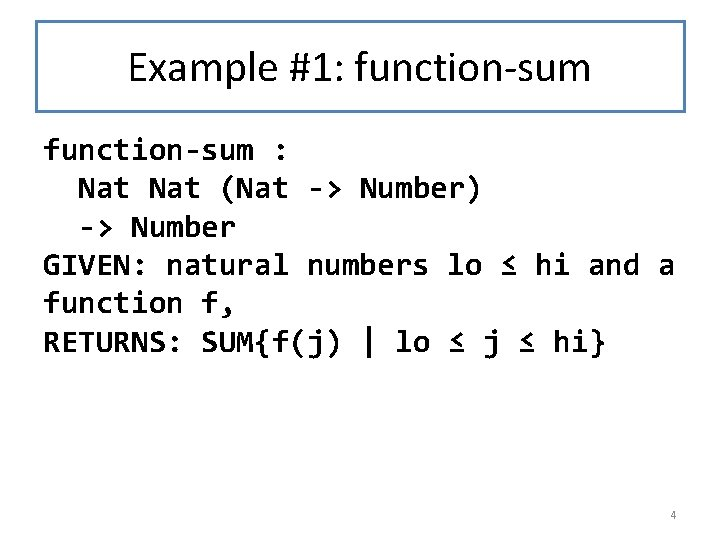Example #1: function-sum : Nat (Nat -> Number) -> Number GIVEN: natural numbers lo ≤ hi and a function f, RETURNS: SUM{f(j) | lo ≤ j ≤ hi} 4Examples/Tests (begin-for-test (check-equal? (function-sum 1 3 (lambda (j) j)) (+ 1 2 3)) (check-equal? (function-sum 1 3 (lambda (j) (+ j 10))) (+ 11 12 13))) 5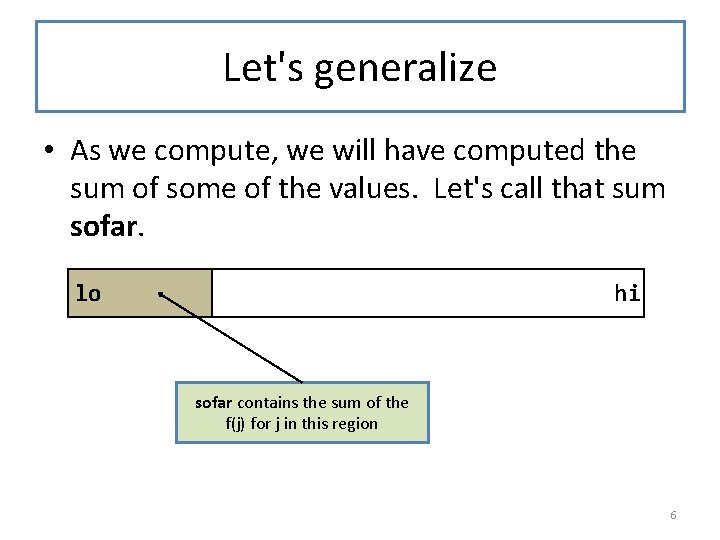Let's generalize • As we compute, we will have computed the sum of some of the values. Let's call that sum sofar. lo hi sofar contains the sum of the f(j) for j in this region 6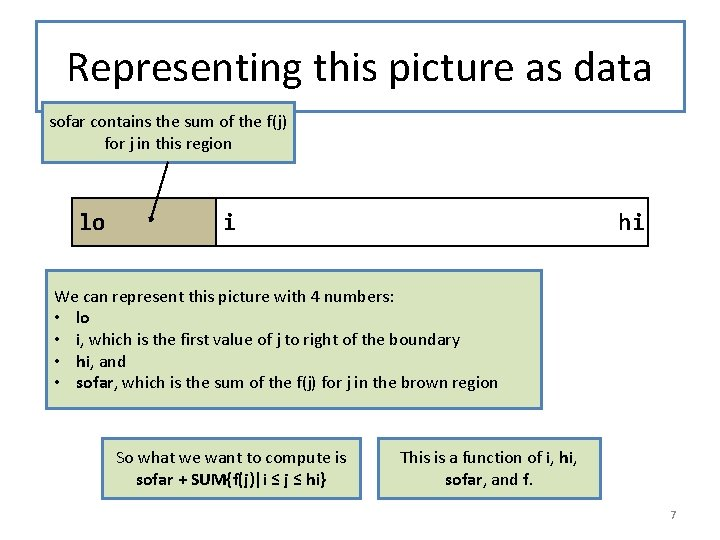Representing this picture as data sofar contains the sum of the f(j) for j in this region lo i hi We can represent this picture with 4 numbers: • lo • i, which is the first value of j to right of the boundary • hi, and • sofar, which is the sum of the f(j) for j in the brown region So what we want to compute is sofar + SUM{f(j)|i ≤ j ≤ hi} This is a function of i, hi, sofar, and f. 7Contract, Purpose Statement, and Examples ; ; ; generalized-function-sum : Nat Number (Nat -> Number) -> Number GIVEN: natural numbers i and hi, a number sofar, and a function f, WHERE: i ≤ hi RETURNS: sofar + SUM{f(j) | i ≤ j ≤ hi} ; ; EXAMPLES/TESTS: (begin-for-test (check-equal? (generalized-function-sum 1 3 17 (lambda (j) j)) (+ 17 (+ 1 2 3))) (check-equal? (generalized-function-sum 1 3 42 (lambda (j) (+ j 10))) (+ 42 (+ 11 12 13)))) 8What do we know about this function? if i = hi, then (generalized-function-sum i hi sofar f) = sofar + SUM{f(j)|i ≤ j ≤ hi} = sofar + SUM{f(j)|hi ≤ j ≤ hi} = (+ sofar (f hi)) = (+ sofar (f i)) 9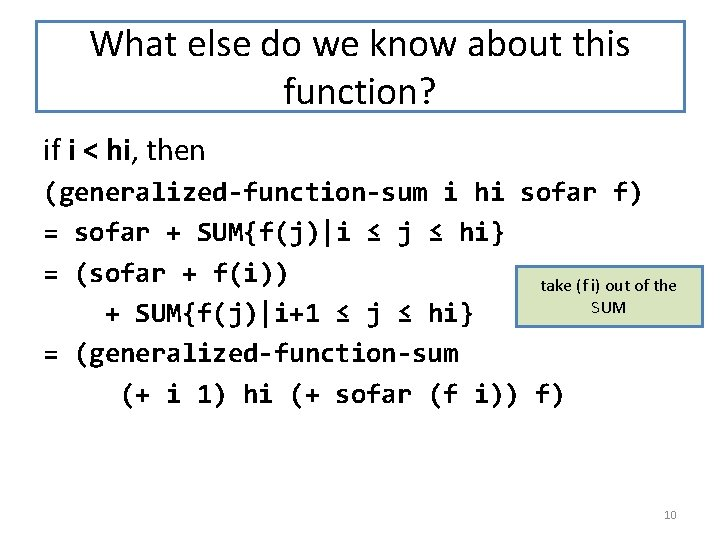What else do we know about this function? if i < hi, then (generalized-function-sum i hi sofar f) = sofar + SUM{f(j)|i ≤ j ≤ hi} = (sofar + f(i)) take (f i) out of the SUM + SUM{f(j)|i+1 ≤ j ≤ hi} = (generalized-function-sum (+ i 1) hi (+ sofar (f i)) f) 10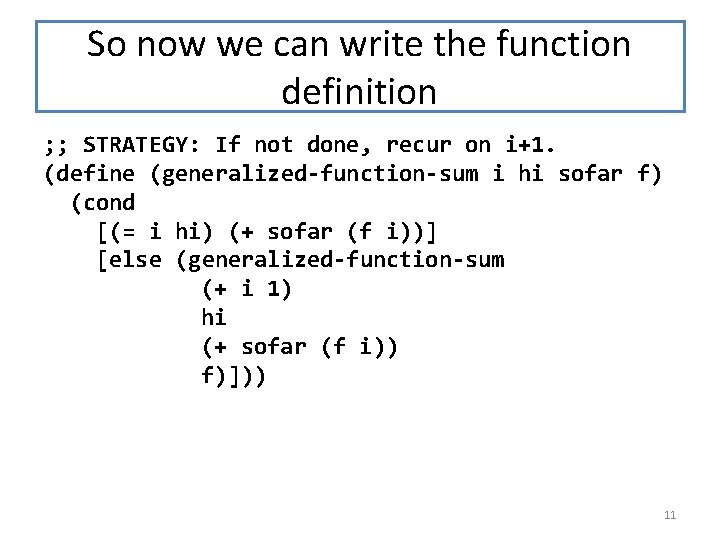So now we can write the function definition ; ; STRATEGY: If not done, recur on i+1. (define (generalized-function-sum i hi sofar f) (cond [(= i hi) (+ sofar (f i))] [else (generalized-function-sum (+ i 1) hi (+ sofar (f i)) f)])) 11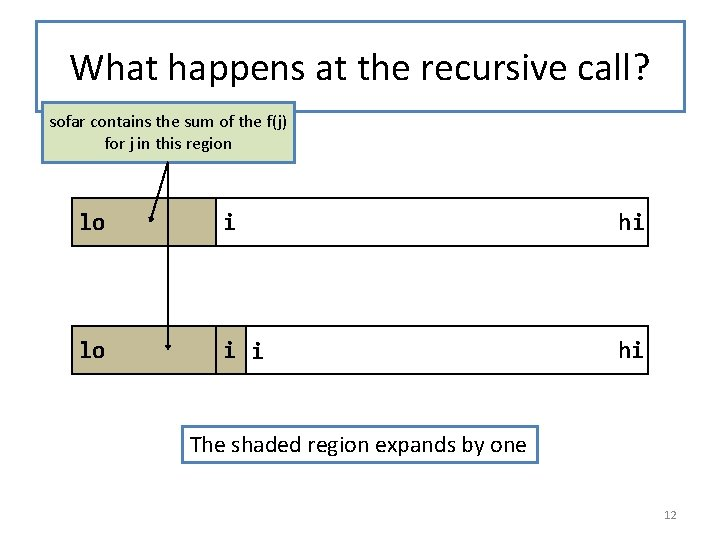What happens at the recursive call? sofar contains the sum of the f(j) for j in this region lo i hi lo i i hi The shaded region expands by one 12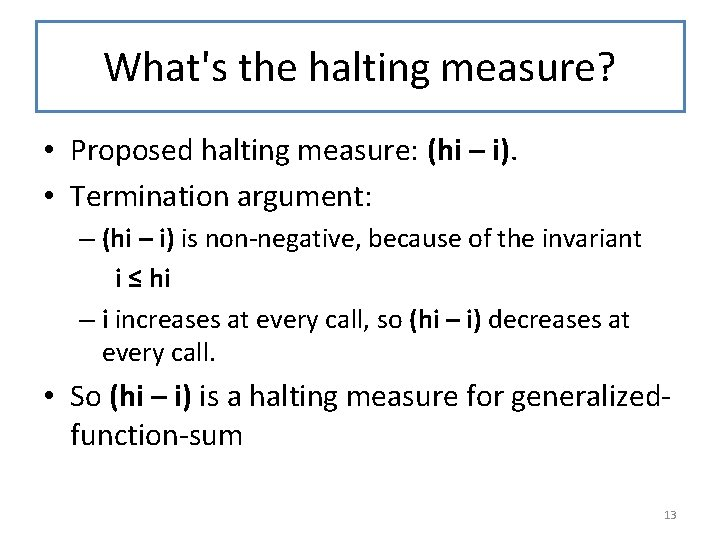What's the halting measure? • Proposed halting measure: (hi – i). • Termination argument: – (hi – i) is non-negative, because of the invariant i ≤ hi – i increases at every call, so (hi – i) decreases at every call. • So (hi – i) is a halting measure for generalizedfunction-sum 13We still need our original function ; ; ; ; function-sum : Nat (Nat -> Number) -> Number GIVEN: natural numbers lo and hi, and a function f WHERE: lo ≤ hi RETURNS: SUM{f(j) | lo ≤ j ≤ hi} STRATEGY: call a more general function (define (function-sum lo hi f) (generalized-function-sum lo hi 0 f)) Just call generalized-function-sum with sofar = 0. 14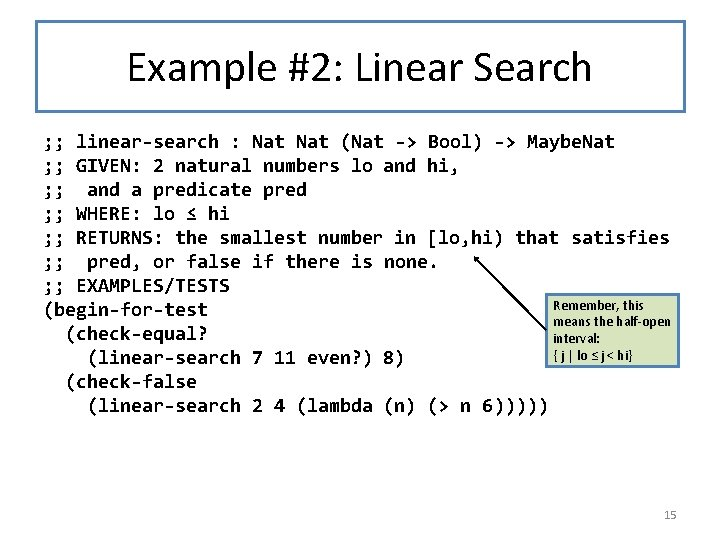Example #2: Linear Search ; ; linear-search : Nat (Nat -> Bool) -> Maybe. Nat ; ; GIVEN: 2 natural numbers lo and hi, ; ; and a predicate pred ; ; WHERE: lo ≤ hi ; ; RETURNS: the smallest number in [lo, hi) that satisfies ; ; pred, or false if there is none. ; ; EXAMPLES/TESTS Remember, this (begin-for-test means the half-open (check-equal? interval: { j | lo ≤ j < hi} (linear-search 7 11 even? ) 8) (check-false (linear-search 2 4 (lambda (n) (> n 6))))) 15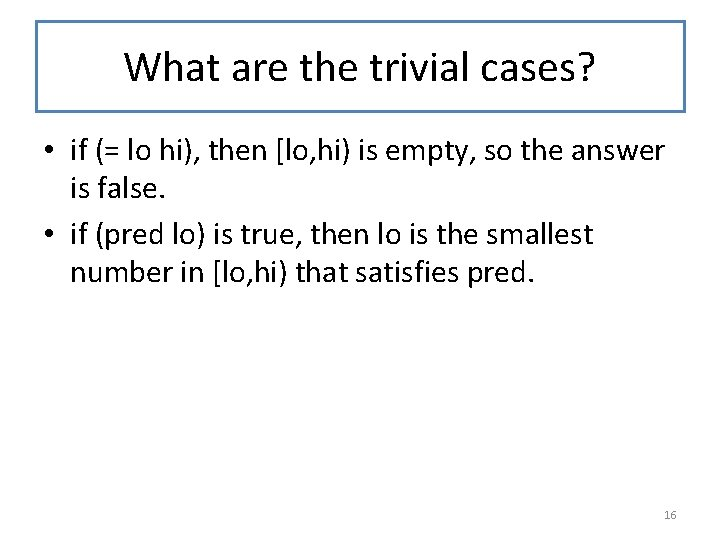What are the trivial cases? • if (= lo hi), then [lo, hi) is empty, so the answer is false. • if (pred lo) is true, then lo is the smallest number in [lo, hi) that satisfies pred. 16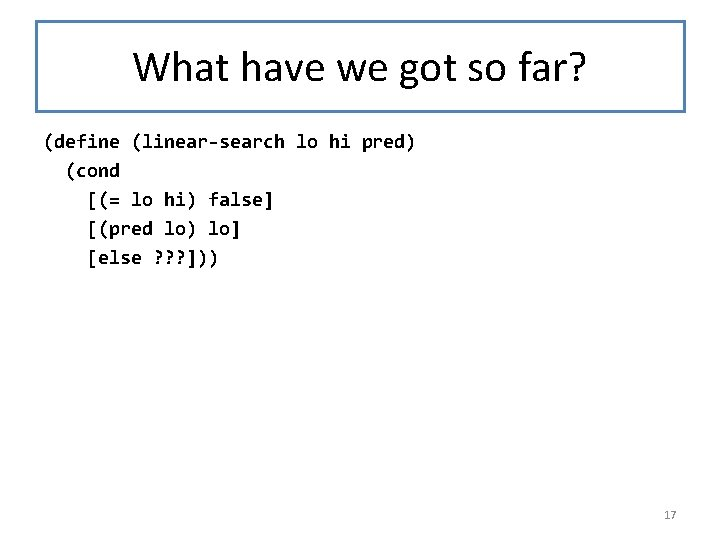What have we got so far? (define (linear-search lo hi pred) (cond [(= lo hi) false] [(pred lo) lo] [else ? ? ? ])) 17What's the non-trivial case? • If (< lo hi) and (pred lo) is false, then the smallest number in [lo, hi) that satisfies pred (if it exists) must be in [lo+1, hi). • So, if (< lo hi) and (pred lo) is false, then (linear-search lo hi pred) = (linear-search (+ lo 1) hi pred) 18Function Definition ; ; STRATEGY: If more to search and not found, then recur ; ; on (+ lo 1) (define (linear-search lo hi pred) (cond [(= lo hi) false] [(pred lo) lo] [else (linear-search (+ lo 1) hi pred)])) 19What's the halting measure? • The invariant tells us that lo ≤ hi, so (- hi lo) is a non-negative integer. • lo increases at every recursive call, so (- hi lo) decreases. • So (- hi lo) is our halting measure. 20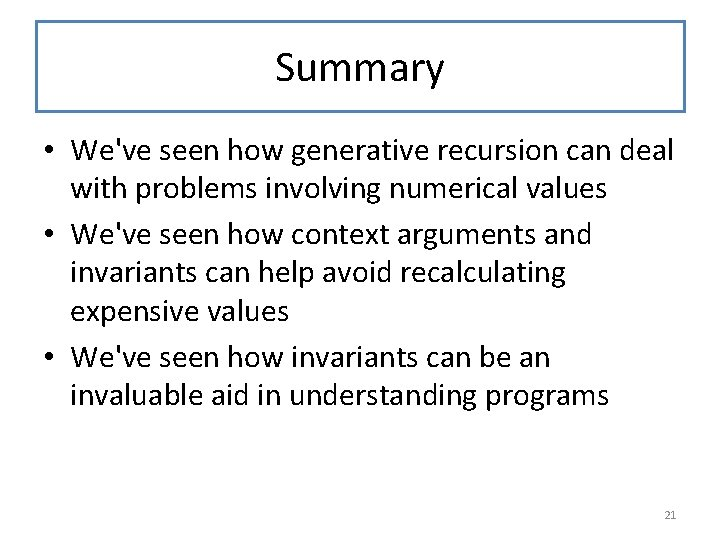Summary • We've seen how generative recursion can deal with problems involving numerical values • We've seen how context arguments and invariants can help avoid recalculating expensive values • We've seen how invariants can be an invaluable aid in understanding programs 21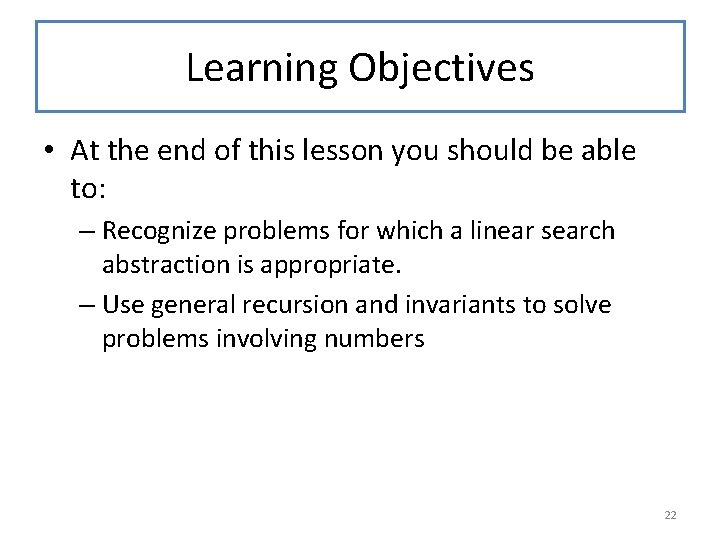Learning Objectives • At the end of this lesson you should be able to: – Recognize problems for which a linear search abstraction is appropriate. – Use general recursion and invariants to solve problems involving numbers 22Next Steps • Study the files 08 -6 -function-sum. rkt and 08 -7 linear-search. rkt • If you have questions about this lesson, ask them on the Discussion Board • Go on to the next lesson 23